WHCSRL 技术网

# 简单谈谈HashMap

## 前言

HashMap 基于哈希表的 Map 接口实现，是以 key-value 存储形式存在，即主要用来存放键值对。HashMap 的实现不是同步的，这意味着它不是线程安全的。它的 key、value 都可以为 null，此外，HashMap 中的映射不是有序的

## hash算法

hash算法又叫摘要算法（Digest），是将给定数据转化为固定长度的不规则值的函数

• 输出值的数据长度固定（同一hash函数下）
• 相同的输入必定得到相同的输出值
• 相似的数据得到的输出值的差距也许会有点大
• 输入不同的数据有可能得到一致的输出值（哈希碰撞，也叫哈希冲突）

``````     //Object类的hashCode方法,返回的是对象的32位jvm内存地址
public native int hashCode();
```12```

String类重写了hashCode()方法

``````    public int hashCode() {
int h = hash;	//Default to 0
if (h == 0 && value.length > 0) {	//private final char value[];
char val[] = value;

for (int i = 0; i < value.length; i++) {
h = 31 * h + val[i];
}
hash = h;
}
return h;
}
```123456789101112```

• 碰撞概率低
• 不能通过哈希值算出原值

### 常用的哈希算法

MD516 bytes128 bits
SHA-120 bytes160 bits
RipeMD-16020 bytes160 bits
SHA-25632 bytes256 bits
SHA-51264 bytes512 bits

## hashmap的数据结构

jdk1.8之前：数组+链表

jdk1.8之后：数组+链表+红黑树

### jdk1.7和jdk1.8的区别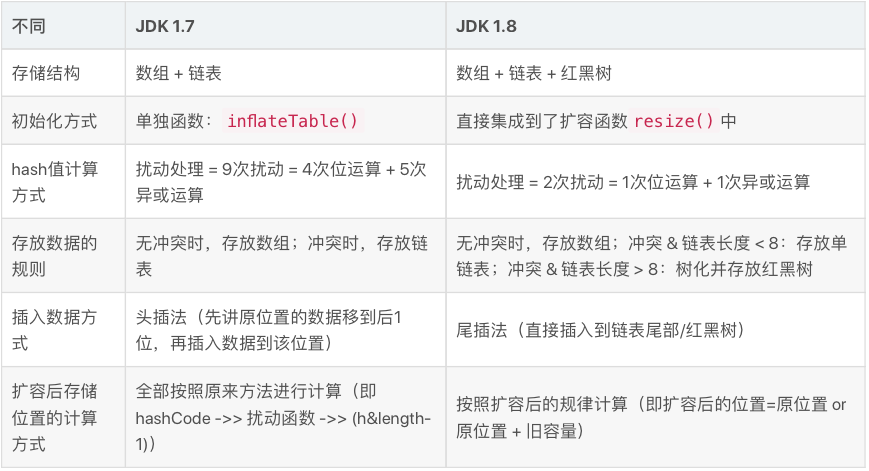### 简单讲解过程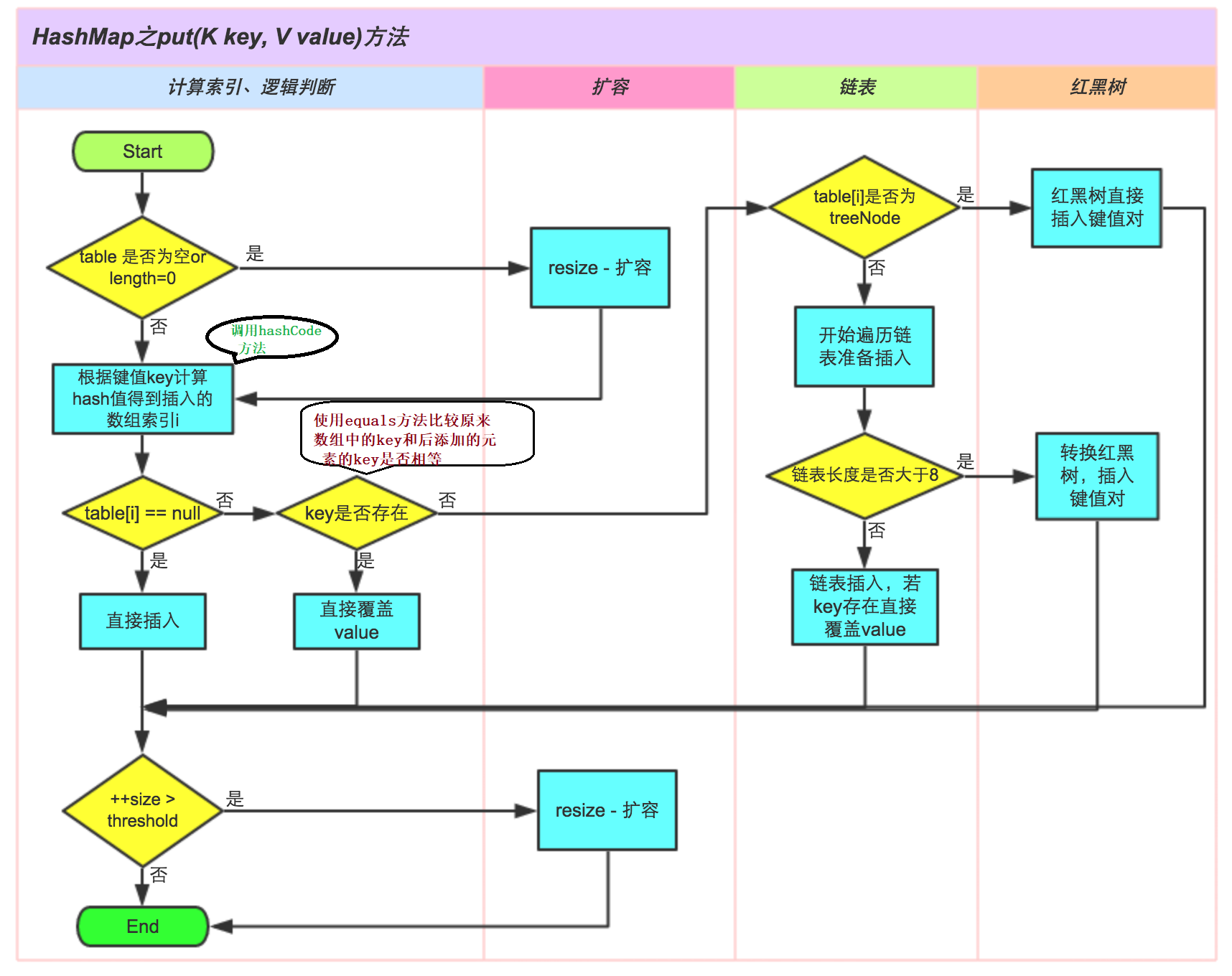### 什么时候扩容？

``````    /**
* The load factor used when none specified in constructor.
*/
static final float DEFAULT_LOAD_FACTOR = 0.75f;

//扩容条件1：table为null 或 tab长度为0
if ((tab = table) == null || (n = tab.length) == 0)
n = (tab = resize()).length;

//扩容条件2：size是否大于了边界值
if (++size > threshold)
resize();
```12345678910111213```

1. size表示hashmap中k-v的实际数量，并不是数组的长度

2. 边界值`threshold` = 容量`DEFAULT_INITIAL_CAPACITY` * 负载因子`DEFAULT_LOAD_FACTOR`

所以达到扩容的阈值点就是16*0.75 = 12

### 扩容源码

hashmap扩容是扩容一倍`newThr = oldThr << 1; // double threshold`（二进制左移一位就是数字翻倍）

cap容量给了老边界值`newCap = oldThr;`

``````    newCap = DEFAULT_INITIAL_CAPACITY;
```12```

ArrayList1010.5倍
Vector1011倍
HashSet160.751 倍
HashMap160.751 倍

### 什么时候转红黑树？

``````	/**
* The value must be greater than 2 and should be at least 8 to mesh with assumptions in
* tree removal about conversion back to plain bins upon shrinkage.
*/
static final int TREEIFY_THRESHOLD = 8;

/**
* The smallest table capacity for which bins may be treeified.
* (Otherwise the table is resized if too many nodes in a bin.)
* Should be at least 4 * TREEIFY_THRESHOLD to avoid conflicts
* between resizing and treeification thresholds.
*/
static final int MIN_TREEIFY_CAPACITY = 64;
```12345678910111213```

``````    if (binCount >= TREEIFY_THRESHOLD - 1) //先判断是否达到链表阈值
treeifyBin(tab, hash);

if (tab == null || (n = tab.length) < MIN_TREEIFY_CAPACITY)//再判断是否达到数组阈值
resize();
```12345```

## HashMap集合类的成员

``````public class HashMap<K,V> extends AbstractMap<K,V>
implements Map<K,V>, Cloneable, Serializable
```12```

`Cloneable`表示可克隆，`Serializable`可序列化

``````public interface Serializable {
}

public interface Cloneable {
}
```12345```

hashmap继承了`AbstractMap<K,V>`，也实现了`Map<K,V>`，可是`AbstractMap<K,V>`也实现了`Map<K,V>`，可能是设计的时候有点bug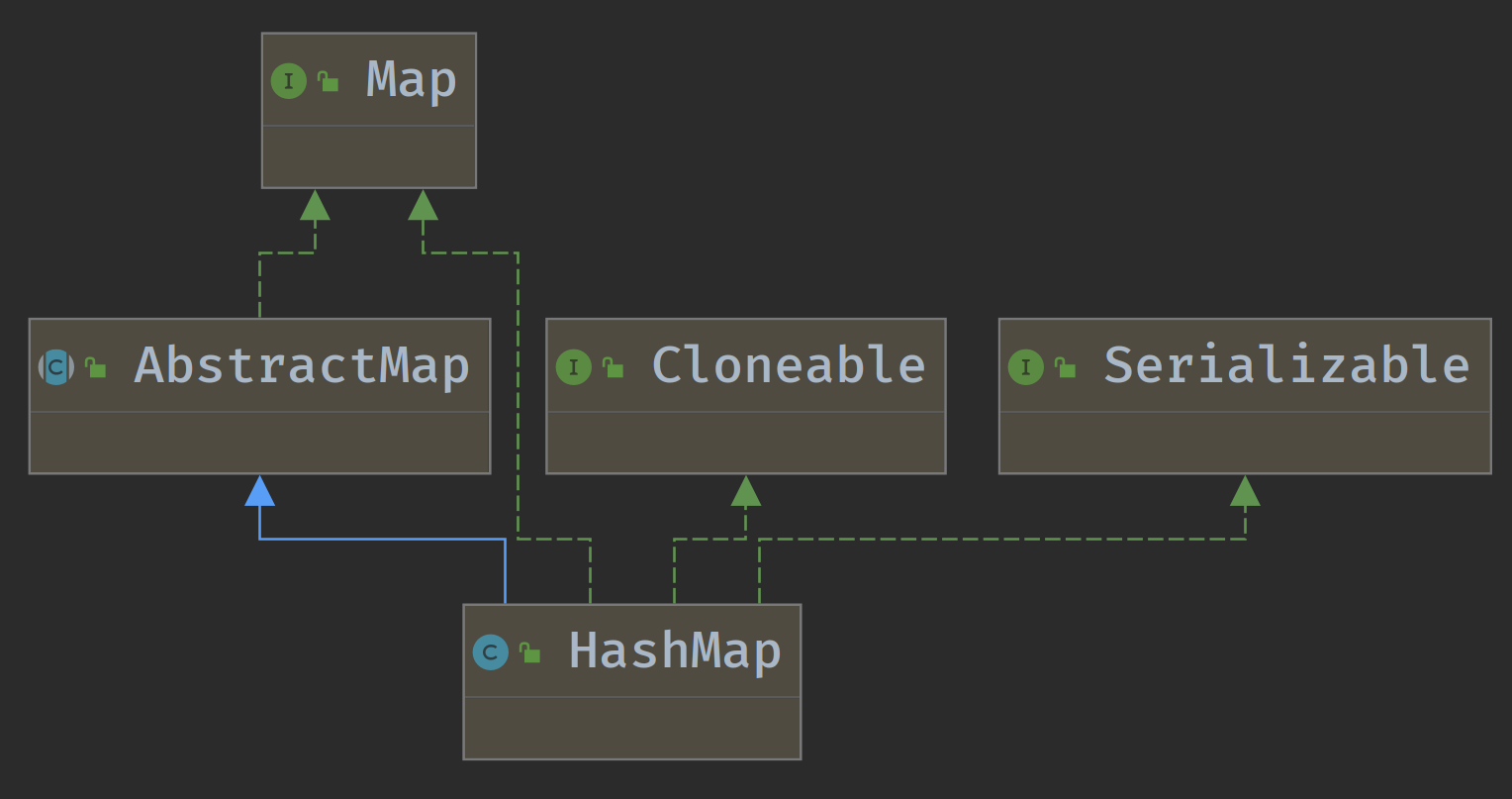### 1.序列化版本号

``````private static final long serialVersionUID = 362498820763181265L;
```1```

• 序列化：将对象写入到IO流中
• 反序列化：从IO流中恢复对象
• 意义：序列化机制允许将实现序列化的Java对象转换位字节序列，这些字节序列可以保存在磁盘上，或通过网络传输，以达到以后恢复成原来的对象。序列化机制使得对象可以脱离程序的运行而独立存在

### 2.集合的初始容量

``````    /**
* The default initial capacity - MUST be a power of two.
*/
static final int DEFAULT_INITIAL_CAPACITY = 1 << 4; // aka 16
```1234```

`默认初始容量 - 必须是 2 的幂`，它为什么必须是2的幂呢？

&：按位与运算，两个当且仅当都为1的时候结果才为1

#### 那我自定义给的容量不是2的N次方呢？

``````    /**
* Returns a power of two size for the given target capacity.
*/
static final int tableSizeFor(int cap) {
int n = cap - 1;
n |= n >>> 1;
n |= n >>> 2;
n |= n >>> 4;
n |= n >>> 8;
n |= n >>> 16;
return (n < 0) ? 1 : (n >= MAXIMUM_CAPACITY) ? MAXIMUM_CAPACITY : n + 1;
}
```123456789101112```

### 3.负载因子（加载因子）

``````static final float DEFAULT_LOAD_FACTOR = 0.75f;
```1```

### 4.集合最大容量

``````static final int MAXIMUM_CAPACITY = 1 << 30;
```1```

### 5.树化阈值（转红黑树的边界值）

``````static final int TREEIFY_THRESHOLD = 8;
static final int MIN_TREEIFY_CAPACITY = 64;
```12```

（其实也需要数组长度大于64，上文也提到了）

``````final void treeifyBin(Node<K,V>[] tab, int hash) {
int n, index; Node<K,V> e;
if (tab == null || (n = tab.length) < MIN_TREEIFY_CAPACITY)
resize();
......
}
```123456```

### 6.取消数化阈值（树转链表）

``````static final int UNTREEIFY_THRESHOLD = 6;
```1```

The value must be greater than 2 and should be at least 8 to mesh with assumptions in tree removal about conversion back to plain bins upon shrinkage.

And when they become too small (due to removal or resizing) they are converted back to plain bins.

## HashMap的构造方法

### 1.默认

``````public HashMap() {
}
```123```

### 2.指定容量

``````public HashMap(int initialCapacity) {
}
```123```

### 3.指定容量和负载因子

``````public HashMap(int initialCapacity, float loadFactor) {
if (initialCapacity < 0)
throw new IllegalArgumentException("Illegal initial capacity: " +
initialCapacity);
if (initialCapacity > MAXIMUM_CAPACITY)
initialCapacity = MAXIMUM_CAPACITY;
throw new IllegalArgumentException("Illegal load factor: " +
this.threshold = tableSizeFor(initialCapacity);
}
```123456789101112```

`this.threshold = tableSizeFor(initialCapacity);`

### 4.指定集合构造器

``````    public HashMap(Map<? extends K, ? extends V> m) {
putMapEntries(m, false);
}

final void putMapEntries(Map<? extends K, ? extends V> m, boolean evict) {
int s = m.size();
if (s > 0) {
if (table == null) { // pre-size
float ft = ((float)s / loadFactor) + 1.0F;
int t = ((ft < (float)MAXIMUM_CAPACITY) ?
(int)ft : MAXIMUM_CAPACITY);
if (t > threshold)
threshold = tableSizeFor(t);
}
else if (s > threshold)
resize();
for (Map.Entry<? extends K, ? extends V> e : m.entrySet()) {
K key = e.getKey();
V value = e.getValue();
putVal(hash(key), key, value, false, evict);
}
}
}
```123456789101112131415161718192021222324```

## HashMap的成员方法

### 1.增加put

• 先通过hash值计算出key映射到哪个桶(数组索引)
• 比对hash，如果桶上没有碰撞冲突，则直接插入
• 如果出现碰撞冲突了，则需要处理冲突
• 如果该桶使用红黑树处理冲突，则调用红黑树的方法插入数据
• 否则采用传统的链式方法插入。如果链的长度达到临界值，则把链转变为红黑树
• 比对key，如果桶中存在重复的键，则为该键替换新值value
• 如果size大于阈值threshold,则进行扩容
``````    //HashMap
public V put(K key, V value) {
//计算key的hash值传给puval方法
return putVal(hash(key), key, value, false, true);
}

static final int hash(Object key) {
int h;
//可以看到，key为null时，hash值为0
//key的hashCode()无符号右移16位 再与 原值  异或^  -> 得到hash值
return (key == null) ? 0 : (h = key.hashCode()) ^ (h >>> 16);
}

//之前提到了hash和数组长度进行 按位与，得到索引
//(tab.length-1)&hash的结果与hash%%tab.length结果一致(length是2的幂次方情况下)
n = tab.length;
tab[i = (n - 1) & hash]
```1234567891011121314151617```

``````        //HashTable put()
if (value == null) {
throw new NullPointerException();
}
int hash = key.hashCode();
```12345```

### 2.核心增加putVal

``````    /**
* Implements Map.put and related methods.
*
* @param hash hash for key
* @param key the key
* @param value the value to put
* @param onlyIfAbsent 如果为true，请不要更改现有值
* @param evict 如果为false，则表处于创建模式
* @return previous value, or null if none
*/
final V putVal(int hash, K key, V value, boolean onlyIfAbsent,
boolean evict)
```123456789101112```

#### 数组table的创建

hashmap的数组是随着new HashMap就被创建了嘛？

``````        if ((tab = table) == null || (n = tab.length) == 0)
n = (tab = resize()).length;
```12```

#### 数组的索引的算法

``````n = tab.length
tab[i = (n - 1) & hash]
```12```

### 3.转化红黑树treeifyBin

``````	//putVal方法中，循环链表，判断count>=链表阈值-1时，进行转化
for (int binCount = 0; ; ++binCount) {
if ((e = p.next) == null) {
p.next = newNode(hash, key, value, null);
if (binCount >= TREEIFY_THRESHOLD - 1) // -1 for 1st
treeifyBin(tab, hash);
break;
}
if (e.hash == hash &&
((k = e.key) == key || (key != null && key.equals(k))))
break;
p = e;
}
```12345678910111213```

#### 什么是2-3-4树？

2-3-4树是四阶的B树(Balance Tree),他属于一种多路查找树,它的结构有以下限制：

• 2-节点：包含1个元素的节点，有2个子节点
• 3-节点：包含2个元素的节点，有3个子节点
• 4-节点：包含3个元素的节点，有4个子节点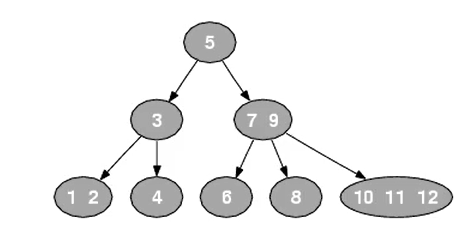2-3-4树的查询操作像普通的二叉搜索树一-样,非常简单,但由于其结点元素数不确定，在一些编程语言中实现起来并不方便， 实现一般使用它的等同——红黑树

#### 2-3-4树和红黑树的关系

• 2节点 - 黑节点
• 3节点 - 左倾/右倾
• 4节点- 中间上移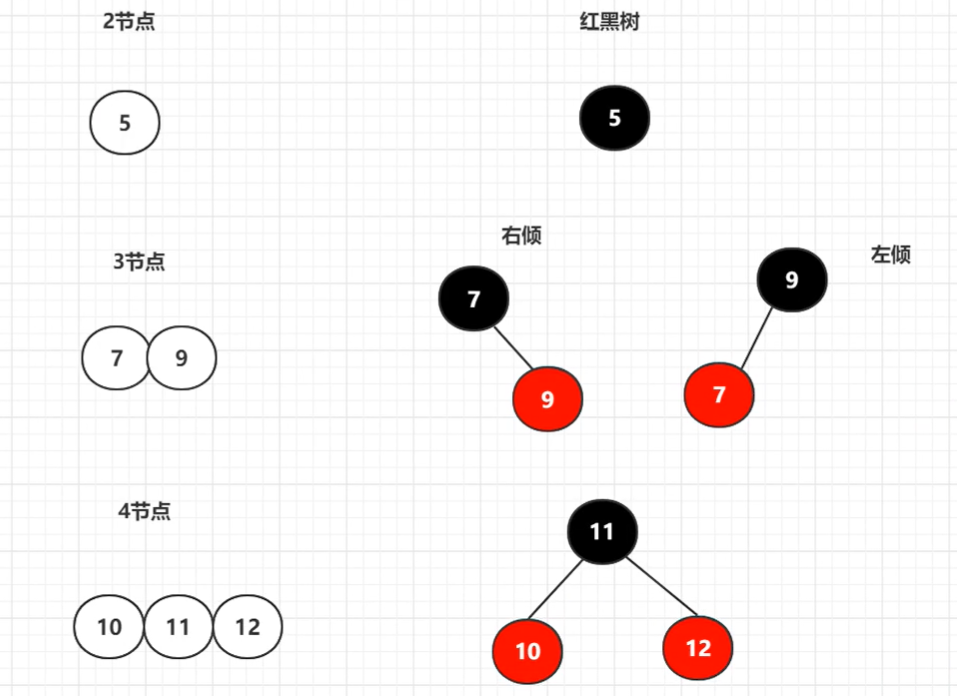#### 什么是红黑树？

• 每个节点要么是黑色，要么是红色

• 根节点是黑色

• 每个叶子节点是黑色

• 一棵树当中没有子结点（即度为0）的结点称为叶子结点

• 每个红色节点的两个子节点一定都是黑色

• 图中红色节点没有子节点是因为默认隐藏掉了为null的黑色节点
• 任意一个节点到每个叶子节点的路径都包含数量相等的黑节点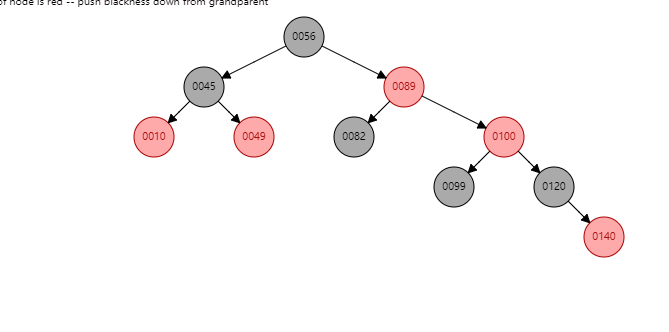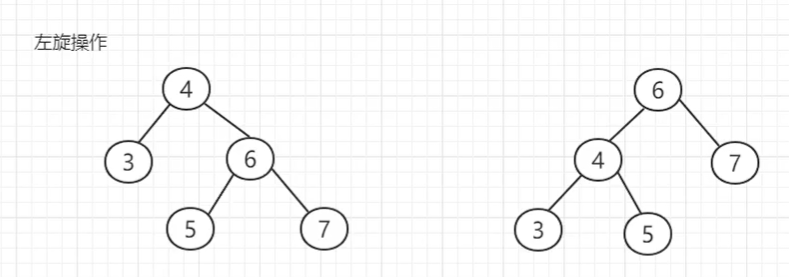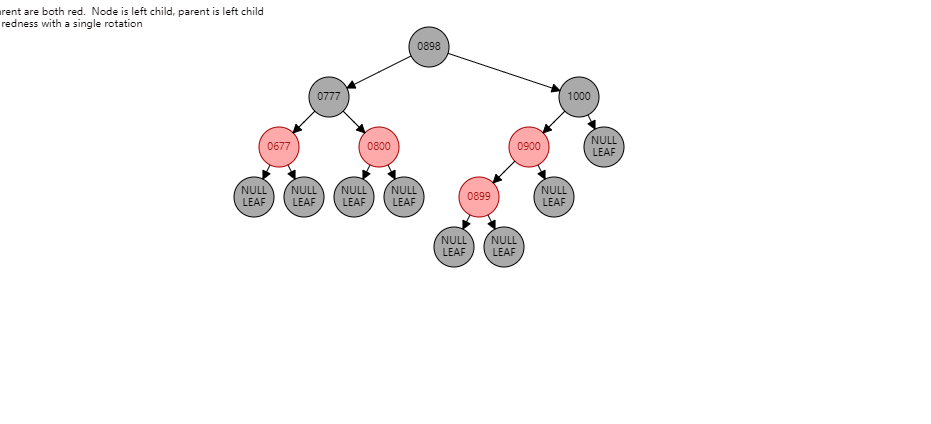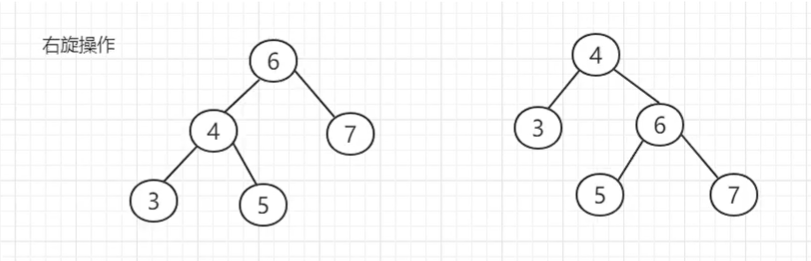#### 红黑树对于插入节点的分析

• 2-3-4树2节点新增一个元素直接合并为3节点
• 红黑树：新增一个红色节点这个红色节点会添加在黑色节点下:不需要调整
• 2-3-4树3节点新增个元素 合并为一个4节点
• 红黑树：就会有6种情况两种(左中右)的不需要调整
• 根左左 根右右 旋转1次
• 根左右 根右左 旋转2次
• 2-3-4树4节点新增一个元素4节点中间元素升级为父节点新增元素和剩下节点合并
• 红黑树：新增节点是红色+爷爷节点是黑色父 节点和叔叔节点为红色
• 调整为爷爷节点变为红色，父亲和叔叔节点变为黑色，如果爷爷节点为root节点则调整为黑色

#### 红黑树对于删除节点的分析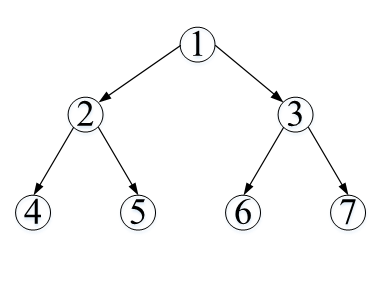1. 删除叶子节点，直接删除
2. 删除的节点有一个子节点，那么用子节点来替代
3. 如果删除的节点右两个子节点，此时需要找到前驱节点或者后继节点替代可以转换为1、2的情况

#### 转换方法treeifyBin

``````    //传入数组tab和hash值用于确定下标
final void treeifyBin(Node<K,V>[] tab, int hash) {
//n是数组长度，index是下标，e是根据hash值从数组中取的节点
int n, index; Node<K,V> e;
//判断数组还有没有容量及是否大于64的数组阈值，没则扩容
if (tab == null || (n = tab.length) < MIN_TREEIFY_CAPACITY)
resize();
//桶中节点不为空则循环转换红黑树节点
else if ((e = tab[index = (n - 1) & hash]) != null) {
//hd链表头结点，tl尾结点
TreeNode<K,V> hd = null, tl = null;
do {
//将普通节点转为树节点
TreeNode<K,V> p = replacementTreeNode(e, null);
if (tl == null)
hd = p;
else {
p.prev = tl;
tl.next = p;
}
tl = p;
} while ((e = e.next) != null);
//链表处理变treeNode后放置回桶中
if ((tab[index] = hd) != null)
//将红黑树节点链表进行平衡操作，才是最终变为红黑树
hd.treeify(tab);
}
}
```12345678910111213141516171819202122232425262728```

``````    TreeNode<K,V> replacementTreeNode(Node<K,V> p, Node<K,V> next) {
return new TreeNode<>(p.hash, p.key, p.value, next);
}
```123```

``````final void treeify(Node<K,V>[] tab) {
TreeNode<K,V> root = null; // 定义树的根节点
for (TreeNode<K,V> x = this, next; x != null; x = next) { // 遍历链表，x指向当前节点、next指向下一个节点
next = (TreeNode<K,V>)x.next; // 下一个节点
x.left = x.right = null; // 设置当前节点的左右节点为空
if (root == null) { // 如果还没有根节点
x.parent = null; // 当前节点的父节点设为空
x.red = false; // 当前节点的红色属性设为false（把当前节点设为黑色）
root = x; // 根节点指向到当前节点
}
else { // 如果已经存在根节点了
K k = x.key; // 取得当前链表节点的key
int h = x.hash; // 取得当前链表节点的hash值
Class<?> kc = null; // 定义key所属的Class
for (TreeNode<K,V> p = root;;) { // 从根节点开始遍历，此遍历没有设置边界，只能从内部跳出
// GOTO1
int dir, ph; // dir 标识方向（左右）、ph标识当前树节点的hash值
K pk = p.key; // 当前树节点的key
if ((ph = p.hash) > h) // 如果当前树节点hash值 大于 当前链表节点的hash值
dir = -1; // 标识当前链表节点会放到当前树节点的左侧
else if (ph < h)
dir = 1; // 右侧

/*
* 如果两个节点的key的hash值相等，那么还要通过其他方式再进行比较
* 如果当前链表节点的key实现了comparable接口，并且当前树节点和链表节点是相同Class的实例，那么通过comparable的方式再比较两者。
* 如果还是相等，最后再通过tieBreakOrder比较一次
*/
else if ((kc == null &&
(kc = comparableClassFor(k)) == null) ||
(dir = compareComparables(kc, k, pk)) == 0)
dir = tieBreakOrder(k, pk);

TreeNode<K,V> xp = p; // 保存当前树节点

/*
* 如果dir 小于等于0 ： 当前链表节点一定放置在当前树节点的左侧，但不一定是该树节点的左孩子，也可能是左孩子的右孩子 或者 更深层次的节点。
* 如果dir 大于0 ： 当前链表节点一定放置在当前树节点的右侧，但不一定是该树节点的右孩子，也可能是右孩子的左孩子 或者 更深层次的节点。
* 如果当前树节点不是叶子节点，那么最终会以当前树节点的左孩子或者右孩子 为 起始节点  再从GOTO1 处开始 重新寻找自己（当前链表节点）的位置
* 如果当前树节点就是叶子节点，那么根据dir的值，就可以把当前链表节点挂载到当前树节点的左或者右侧了。
* 挂载之后，还需要重新把树进行平衡。平衡之后，就可以针对下一个链表节点进行处理了。
*/
if ((p = (dir <= 0) ? p.left : p.right) == null) {
x.parent = xp; // 当前链表节点 作为 当前树节点的子节点
if (dir <= 0)
xp.left = x; // 作为左孩子
else
xp.right = x; // 作为右孩子
root = balanceInsertion(root, x); // 重新平衡
break;
}
}
}
}

// 把所有的链表节点都遍历完之后，最终构造出来的树可能经历多个平衡操作，根节点目前到底是链表的哪一个节点是不确定的
// 因为我们要基于树来做查找，所以就应该把 tab[N] 得到的对象一定根是节点对象，而目前只是链表的第一个节点对象，所以要做相应的处理。
//把红黑树的根节点设为  其所在的数组槽 的第一个元素
//首先明确：TreeNode既是一个红黑树结构，也是一个双链表结构
//这个方法里做的事情，就是保证树的根节点一定也要成为链表的首节点
moveRootToFront(tab, root);
}
```1234567891011121314151617181920212223242526272829303132333435363738394041424344454647484950515253545556575859606162```

### 4.扩容resize

#### 容量翻倍

``````	final Node<K,V>[] resize() {
//获取扩容之前的元素列表
Node<K,V>[] oldTab = table;
//获取之前的列表长度
int oldCap = (oldTab == null) ? 0 : oldTab.length;
int oldThr = threshold;
int newCap, newThr = 0;

if (oldCap > 0) {
//如果原来的容量已经大于了最大容量,则把threshold设置成int的最大值
if (oldCap >= MAXIMUM_CAPACITY) {
threshold = Integer.MAX_VALUE;
return oldTab;
}
//否则,新的容量,扩充为原来的一杯oldCap << 1 向左位移1为,相当于oldCap * 2
else if ((newCap = oldCap << 1) < MAXIMUM_CAPACITY &&
oldCap >= DEFAULT_INITIAL_CAPACITY)
newThr = oldThr << 1; // double threshold
}
else if (oldThr > 0) // initial capacity was placed in threshold
newCap = oldThr;

//如果走下面这个else,则说明是第一次put元素,也是第一次进行扩展
else {               // zero initial threshold signifies using defaults
newCap = DEFAULT_INITIAL_CAPACITY;

//扩容 = 扩容因子 * 容量,DEFAULT_INITIAL_CAPACITY 默认是16,DEFAULT_LOAD_FACTOR默认是0.75
}
```123456789101112131415161718192021222324252627282930```

#### 重新计算，并旧转新

``````
@SuppressWarnings({"rawtypes","unchecked"})
Node<K,V>[] newTab = (Node<K,V>[])new Node[newCap];
table = newTab;

//到此为止,如果是第一次扩容的时候,也就是原来没有元素,下面的代码不会运行
//如果原来有元素,则将原来的元素,进行放到新扩容的数组里面
if (oldTab != null) {
//循环原来的列表，将旧数组放置新数组中
for (int j = 0; j < oldCap; ++j) {
Node<K,V> e;
if ((e = oldTab[j]) != null) {
oldTab[j] = null;
//如果列表里面只有一个元素,直接将e的key的hash与新容量重新计算下标

if (e.next == null)
newTab[e.hash & (newCap - 1)] = e;
//如果是红黑树,则进行红黑树的复制
else if (e instanceof TreeNode)
((TreeNode<K,V>)e).split(this, newTab, j, oldCap);
else {
/*
这一块是处理原来的HashMap冲突的，因为容量扩容了
原来通过 & 运算出来的hash冲突的key,与新的容量 & 可能位置会发生改变
比如之前的冲突的两个key （k1,k2）的hash 分别为 1101，11101
初始化默认的容量是16 二进制就是 10000， 16-1的二进制就是 1111 与key进行& 运算结果都是 1101，但是与11111(36-1)进行 一个是1101 另一是11101
所以需要重新计算下标，通过与oldCap 进行 & 运算 分为低位和高位，因为和oldCap &运算只有两种结果，一种是0 另一种是oldCap
将结果=0的设置成低位，将等于oldCap的保存到高位，
低位的hash值肯定小于oldCap，所以下标还是在原来的位置
高位的hash一定大于oldCap，在二进制最左至少会多一个1（有可能还有前面还有其他二进制数字，但运算结果都是一样的）计算正好是原来位置加上新的容量
*/
//低位位链表头和尾
Node<K,V> loHead = null, loTail = null;
//高位链表头和尾
Node<K,V> hiHead = null, hiTail = null;
Node<K,V> next;
do {
//获取当前节点的下一个元素
next = e.next;

if ((e.hash & oldCap) == 0) {
if (loTail == null)
else
//如果不是第一个元素,就把他加到后面
loTail.next = e;
loTail = e;
}
//高位节点的流程
else {
if (hiTail == null)
else
hiTail.next = e;
hiTail = e;
}
} while ((e = next) != null);
if (loTail != null) {
loTail.next = null;
//将原来下标指向低位的链表
}
if (hiTail != null) {
hiTail.next = null;
//下标 j + oldCap 计算出来的新下标正好是原来位置索引加上新的容量
}
}
}
}
}
return newTab;
}
```1234567891011121314151617181920212223242526272829303132333435363738394041424344454647484950515253545556575859606162636465666768697071727374```

#### 扩容方法中JDK8对JDK7的优化

jdk7中，所有元素按照索引算法全部重新计算效率比较低

``````//jdk1.7
void resize(int newCapacity) {
Entry[] oldTable = table;
int oldCapacity = oldTable.length;
//判断是否有超出扩容的最大值，如果达到最大值则不进行扩容操作
if (oldCapacity == MAXIMUM_CAPACITY) {
threshold = Integer.MAX_VALUE;
return;
}

Entry[] newTable = new Entry[newCapacity];
// transfer()方法把原数组中的值放到新数组中
transfer(newTable, initHashSeedAsNeeded(newCapacity));
//设置hashmap扩容后为新的数组引用
table = newTable;
//设置hashmap扩容新的阈值
threshold = (int)Math.min(newCapacity * loadFactor, MAXIMUM_CAPACITY + 1);
}

void transfer(Entry[] newTable, boolean rehash) {
int newCapacity = newTable.length;
for (Entry<K,V> e : table) {
while(null != e) {
Entry<K,V> next = e.next;
if (rehash) {
e.hash = null == e.key ? 0 : hash(e.key);
}
//通过key值的hash值和新数组的大小算出在当前数组中的存放位置
int i = indexFor(e.hash, newCapacity);
e.next = newTable[i];
newTable[i] = e;
e = next;
}
}
}
```1234567891011121314151617181920212223242526272829303132333435```

jdk8中，在扩充HashMap的时候,不需要重新计算hash，只需要看看原来的hash值新增的那个bit是1还是0就行

`e.hash & oldCap`进行位运算，判断结果

• 是0的话，索引没变：原位置 `newTab[j] = loHead;`
• 是1的话，索引变成：原索引+oldCap(原位置+旧容量) `newTab[j + oldCap] = hiHead;`

rehash之后不会出现更严重的hash冲突，均匀的把之前的冲突的节点分散到新的桶中了。

``````    //jdk1.8

if ((e.hash & oldCap) == 0) {
if (loTail == null)
else
loTail.next = e;
loTail = e;
}
else {
if (hiTail == null)
else
hiTail.next = e;
hiTail = e;
}
//扩容后的位置=原位置
if (loTail != null) {
loTail.next = null;
}
//扩容后的位置 = 原位置 + 旧容量
if (hiTail != null) {
hiTail.next = null;
}
```1234567891011121314151617181920212223242526```

### 5.删除remove

``````    public V remove(Object key) {
Node<K,V> e;
return (e = removeNode(hash(key), key, null, false, true)) == null ?
null : e.value;
}
```12345```

### 6.核心删除removeNode

``````/**
* 方法为final，不可被覆写，子类可以通过实现afterNodeRemoval方法来增加自己的处理逻辑（解析中有描述）
*
* @param hash key的hash值，该值是通过hash(key)获取到的
* @param key 要删除的键值对的key
* @param value 要删除的键值对的value，该值是否作为删除的条件取决于matchValue是否为true
* @param matchValue 如果为true，则当key对应的键值对的值equals(value)为true时才删除；否则不关心value的值
* @param movable 删除后是否移动节点，如果为false，则不移动
* @return 返回被删除的节点对象，如果没有删除任何节点则返回null
*/
final Node<K,V> removeNode(int hash, Object key, Object value,
boolean matchValue, boolean movable) {
Node<K,V>[] tab; Node<K,V> p; int n, index; // 声明节点数组、当前节点、数组长度、索引值
/*
* 如果 节点数组tab不为空、数组长度n大于0、根据hash定位到的节点对象p（该节点为 树的根节点 或 链表的首节点）不为空
* 需要从该节点p向下遍历，找到那个和key匹配的节点对象
*/
if ((tab = table) != null && (n = tab.length) > 0 &&
(p = tab[index = (n - 1) & hash]) != null) {
Node<K,V> node = null, e; K k; V v; // 定义要返回的节点对象，声明一个临时节点变量、键变量、值变量

// 如果当前节点的键和key相等，那么当前节点就是要删除的节点，赋值给node
if (p.hash == hash &&
((k = p.key) == key || (key != null && key.equals(k))))
node = p;

/*
* 到这一步说明首节点没有匹配上，那么检查下是否有next节点
* 如果没有next节点，就说明该节点所在位置上没有发生hash碰撞, 就一个节点并且还没匹配上，也就没得删了，最终也就返回null了
* 如果存在next节点，就说明该数组位置上发生了hash碰撞，此时可能存在一个链表，也可能是一颗红黑树
*/
else if ((e = p.next) != null) {
// 如果当前节点是TreeNode类型，说明已经是一个红黑树，那么调用getTreeNode方法从树结构中查找满足条件的节点
if (p instanceof TreeNode)
node = ((TreeNode<K,V>)p).getTreeNode(hash, key);
// 如果不是树节点，那么就是一个链表，只需要从头到尾逐个节点比对即可
else {
do {
// 如果e节点的键是否和key相等，e节点就是要删除的节点，赋值给node变量，调出循环
if (e.hash == hash &&
((k = e.key) == key ||
(key != null && key.equals(k)))) {
node = e;
break;
}

// 走到这里，说明e也没有匹配上
p = e; // 把当前节点p指向e，这一步是让p存储的永远下一次循环里e的父节点，如果下一次e匹配上了，那么p就是node的父节点
} while ((e = e.next) != null); // 如果e存在下一个节点，那么继续去匹配下一个节点。直到匹配到某个节点跳出 或者 遍历完链表所有节点
}
}

/*
* 如果node不为空，说明根据key匹配到了要删除的节点
* 如果不需要对比value值  或者  需要对比value值但是value值也相等
* 那么就可以删除该node节点了
*/
if (node != null && (!matchValue || (v = node.value) == value ||
(value != null && value.equals(v)))) {
if (node instanceof TreeNode) // 如果该节点是个TreeNode对象，说明此节点存在于红黑树结构中，调用removeTreeNode方法（该方法单独解析）移除该节点
((TreeNode<K,V>)node).removeTreeNode(this, tab, movable);
else if (node == p) // 如果该节点不是TreeNode对象，node == p 的意思是该node节点就是首节点
tab[index] = node.next; // 由于删除的是首节点，那么直接将节点数组对应位置指向到第二个节点即可
else // 如果node节点不是首节点，此时p是node的父节点，由于要删除node，所有只需要把p的下一个节点指向到node的下一个节点即可把node从链表中删除了
p.next = node.next;
++modCount; // HashMap的修改次数递增
--size; // HashMap的元素个数递减
afterNodeRemoval(node); // 调用afterNodeRemoval方法，该方法HashMap没有任何实现逻辑，目的是为了让子类根据需要自行覆写
return node;
}
}
return null;
}
```12345678910111213141516171819202122232425262728293031323334353637383940414243444546474849505152535455565758596061626364656667686970717273```

### 7.获取get

``````    public V get(Object key) {
Node<K,V> e;
return (e = getNode(hash(key), key)) == null ? null : e.value;
}
```1234```

### 8.核心获取getNode

``````    if (first instanceof TreeNode)
return ((TreeNode<K,V>)first).getTreeNode(hash, key);
```12```

``````    do {
if (e.hash == hash &&
((k = e.key) == key || (key != null && key.equals(k))))
return e;
} while ((e = e.next) != null);
```12345```

``````    final Node<K,V> getNode(int hash, Object key) {
Node<K,V>[] tab; Node<K,V> first, e; int n; K k;
if ((tab = table) != null && (n = tab.length) > 0 &&
(first = tab[(n - 1) & hash]) != null) {
if (first.hash == hash && // always check first node
((k = first.key) == key || (key != null && key.equals(k))))
return first;
if ((e = first.next) != null) {
if (first instanceof TreeNode)
return ((TreeNode<K,V>)first).getTreeNode(hash, key);
do {
if (e.hash == hash &&
((k = e.key) == key || (key != null && key.equals(k))))
return e;
} while ((e = e.next) != null);
}
}
return null;
}
```12345678910111213141516171819```

## 遍历hashmap的5种方式

### 1.循环遍历map自带的entrySet中的entry

map的entrySet()方法可以返回`Set<Map.Entry<K, V>> entrySet();`

``````    Map<Integer, Integer> map = new HashMap<Integer, Integer>();
map.put(1, 10);
map.put(2, 20);

Set<Map.Entry<Integer, Integer>> entries = map.entrySet();
for (Map.Entry<Integer, Integer> entry : entries) {
System.out.println("key ="+entry.getKey()+" value ="+entry.getValue());
}
```12345678```

### 2.ForEach迭代键值对方式

`map.keySet()``map.values()`包含了所有的key和value，直接遍历即可

``````    Map<Integer, Integer> map = new HashMap<Integer, Integer>();
map.put(1, 10);
map.put(2, 20);

// 迭代键
for (Integer key : map.keySet()) {
System.out.println("Key = " + key);
}

// 迭代值
for (Integer value : map.values()) {
System.out.println("Value = " + value);
}
```12345678910111213```

### 3.带泛型的map的entrySet的迭代器进行遍历

`map.entrySet()`返回的set可以使用iterator()方法得到迭代器

`entries.next()`得到entry，和第一个方法一样getKey和getValue就行

``````    Map<Integer, Integer> map = new HashMap<Integer, Integer>();
map.put(1, 10);
map.put(2, 20);

Iterator<Map.Entry<Integer, Integer>> entries = map.entrySet().iterator();
while (entries.hasNext()) {
Map.Entry<Integer, Integer> entry = entries.next();
System.out.println("Key = " + entry.getKey() + ", Value = " + entry.getValue());
}
```123456789```

### 4.不带泛型的map的entrySet的迭代器进行遍历

``````    Map map = new HashMap();
map.put(1, 10);
map.put(2, 20);

Iterator<Map.Entry> entries = map.entrySet().iterator();
while (entries.hasNext()) {
Map.Entry entry = (Map.Entry) entries.next();
Integer key = (Integer) entry.getKey();
Integer value = (Integer) entry.getValue();
System.out.println("Key = " + key + ", Value = " + value);
}
```1234567891011```

### 5.通过Java8 Lambda表达式遍历

``````    Map<Integer, Integer> map = new HashMap<Integer, Integer>();
map.put(1, 10);
map.put(2, 20);
map.forEach((k, v) -> System.out.println("key: " + k + " value:" + v));
```1234```